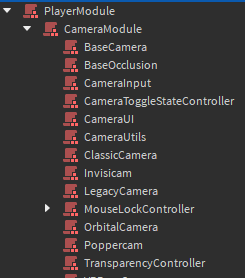# How would I go about creating an "Offset angle" for the camera?

1. What do you want to achieve?
I want to make a camera bobbing effect that goes up and down when moving.

2. What is the issue?
When I change the CFrame angle of the camera, it proceeds to go up and change the direction of my character.
Up and down Gif: https://gyazo.com/d7c1363642e946261c84be8bba270470
Example if it was sideways: https://gyazo.com/29b5c4fdba132cdfcfccb56db4ed89f1

3. What solutions have you tried so far?
I thought about changing the way the Roblox camera module works but…… Let’s just say it’s pretty complicated.
However, I did try to look into those scripts, and from what I’ve seen it compiles all CFrames together(?)
I did try keeping the camera CFrame locked to the HumanoidRootPart’s position with a Vector3 offset, so this is sorta how I want it to be: https://gyazo.com/cc6ce954a8e4213cec6150e1186dd3a7

This is my script currently.
``````--// Services //--
local RunService	=	game:GetService("RunService")
local Players		=	game:GetService("Players")

--// Variables //--

--// Player
local Player	=	Players.LocalPlayer
local Character	=	Player.Character
local Humanoid	=	Character:WaitForChild("Humanoid")
local Camera	=	workspace.CurrentCamera

--// Bobbing
local Time		=	0
local Intensity	=	0
local Speed		=	0

local Offset_Pos = {
X = 0,
Y = 0,
Z = 0
}

local Offset_Ang = {
X = 0,
Y = 0,
Z = 0
}

--// Functions //--
Humanoid.Running:Connect(function(NewSpeed)
Speed = NewSpeed
end)

RunService.RenderStepped:Connect(function(DeltaTime)

Intensity = math.clamp(Intensity+((Humanoid.MoveDirection.Magnitude > 0 and 1 or 0)-Intensity)*(DeltaTime*4),0,1)

Time += Speed/2*DeltaTime*(Humanoid:GetState() == Enum.HumanoidStateType.Freefall and 0.25 or 1)

Offset_Pos.X = math.sin(Time)*0.25
Offset_Pos.Y = math.abs(math.cos(Time))*0.75
Offset_Pos.Y -= Offset_Pos.Y*0.5

Offset_Ang.X = math.abs(math.sin(Time))
Offset_Ang.Z = -(math.sin(Time)*0.5)

Humanoid.CameraOffset = Vector3.new(
Offset_Pos.X*Intensity,
Offset_Pos.Y*Intensity,
Offset_Pos.Z*Intensity
)

Camera.CFrame *= CFrame.Angles(
)
end)
``````

All I want to ask is, is there a way to create an “offset angle” for the camera?
Imagine there’s the original CFrame, where the camera is originally angled at, and a “fake” CFrame.
Then a script combines them together and changes the actual CFrame of the camera.
Example:

``````local FinalCFrame = CFrame.new()
for i,cFrame in pairs(Camera:GetAttributes()) do
FinalCFrame *= cFrame
end

Camera.CFrame = FinalCFrame
``````

I’m sorry if this is a lot to ask, I couldn’t find a solution, maybe my question is vague.
Either way, thanks for reading and I hope someone understandsIn your script, you are using the CFrame of the camera to create a bobbing effect when the character moves. However, this leads to the character’s direction changing. You need to separate the camera’s position and the character’s direction. You can do this by using the Camera.CFrame property, which determines the position and direction of the camera, and the HumanoidRootPart.CFrame property, which determines the position and direction of the character. You can create an offset for the camera using a Vector3 and add it to the HumanoidRootPart.CFrame to achieve the desired camera bobbing effect.

That’s not exactly what I’m aiming for, what I want is that the camera angle will stay relative to the original CFrame and then add the new “fake” angle.

Example:
The camera is looking at a tree.
When the player moves, the camera is still looking at the tree, but the bobbing effect is shown.
However, the bobbing effect only makes the camera change its offset from the tree, but it’s still originally looking at it.

Based off of what I learned previously, I came up with this. The bobbing effect is there, and it does not change the character’s direction. I do not know if this helps.

``````RunService.RenderStepped:Connect(function()
local offset

if humanoid.MoveDirection.Magnitude > 0 then
local y = math.abs(math.sin(tick() * --[[speed]])) * --[[intensity]]

offset = HUM_OFFSET:Lerp(Vector3.new(0, y, 0), --[[smoothness]])
else
offset = HUM_OFFSET
end

humanoid.CameraOffset = offset
end)
``````

1 Like

This changes the Humanoid CameraOffset, not the camera angle.
I still appreciate the help though :))

That is intended,

I believe you can do this by combining these two topics together, a camera script to get the camera rotation without the offsets and additional effects and a custom movement script to make the character move in the direction of the camera without the offsets.

This is my inital idea will look into it further as its interesting.

[CameraService Demo.rbxl (135.7 КБ)](Camera Service Demo - Roblox)

Works exactly how I wanted! I used the first technique (use an old and new offsets for the camera), however I changed it so it’ll work betterCurrent script
``````--// Services //--
local RunService	=	game:GetService("RunService")
local Players		=	game:GetService("Players")

--// Variables //--

--// Player
local Player	=	Players.LocalPlayer
local Character	=	Player.Character
local Humanoid	=	Character:WaitForChild("Humanoid")
local Camera	=	workspace.CurrentCamera

--// Bobbing
local Time		=	0
local Intensity	=	0
local Speed		=	0

local Offset_Pos = {
X = 0,
Y = 0,
Z = 0
}

local Offset_Ang = {
X = 0,
Y = 0,
Z = 0
}

local OldAngle = CFrame.new()
local NewAngle = CFrame.new()

--// Functions //--
Humanoid.Running:Connect(function(NewSpeed)
Speed = NewSpeed
end)

RunService.RenderStepped:Connect(function(DeltaTime)
local NewIntensity = (Humanoid.MoveDirection.Magnitude > 0 and 1 or 0)*(Speed/18)
Intensity = math.clamp(Intensity+(NewIntensity-Intensity)*(DeltaTime*4),0,1)

Time += Speed*0.5*DeltaTime*(Humanoid:GetState() == Enum.HumanoidStateType.Freefall and 0.25 or 1)

Offset_Pos.X = math.sin(Time)*0.25
Offset_Pos.Y = math.abs(math.cos(Time))*0.75
Offset_Pos.Y -= Offset_Pos.Y*0.5

Offset_Ang.X = math.abs(math.cos(Time)*0.25)
Offset_Ang.Z = -(math.sin(Time)*0.5)

Humanoid.CameraOffset = Vector3.new(
Offset_Pos.X*Intensity,
Offset_Pos.Y*Intensity,
Offset_Pos.Z*Intensity
)

local CamCFrame = Camera.CFrame*OldAngle:Inverse()

NewAngle = CFrame.Angles(